# C继承，万字笔记简单易懂

时间：2022-05-11 15:51:01  阅读量：16  评论数：0  作者：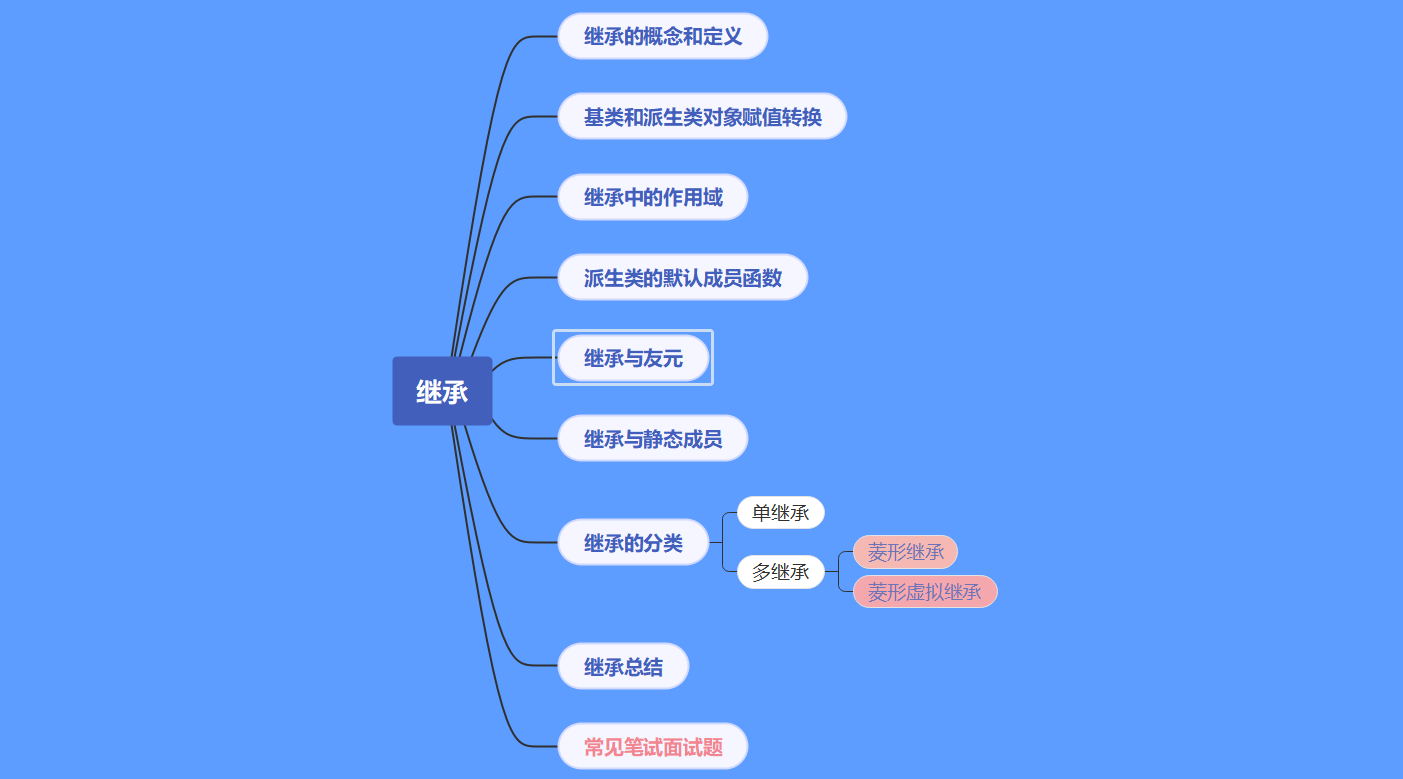# 🌌继承的定义

`简单来说`继承本质上就是`代码复用`，而且是`类层次的复用`，子类继承父类,子类可以复用父类的成员（成员变量和成员函数）。基类又称为父类，派生类又称为子类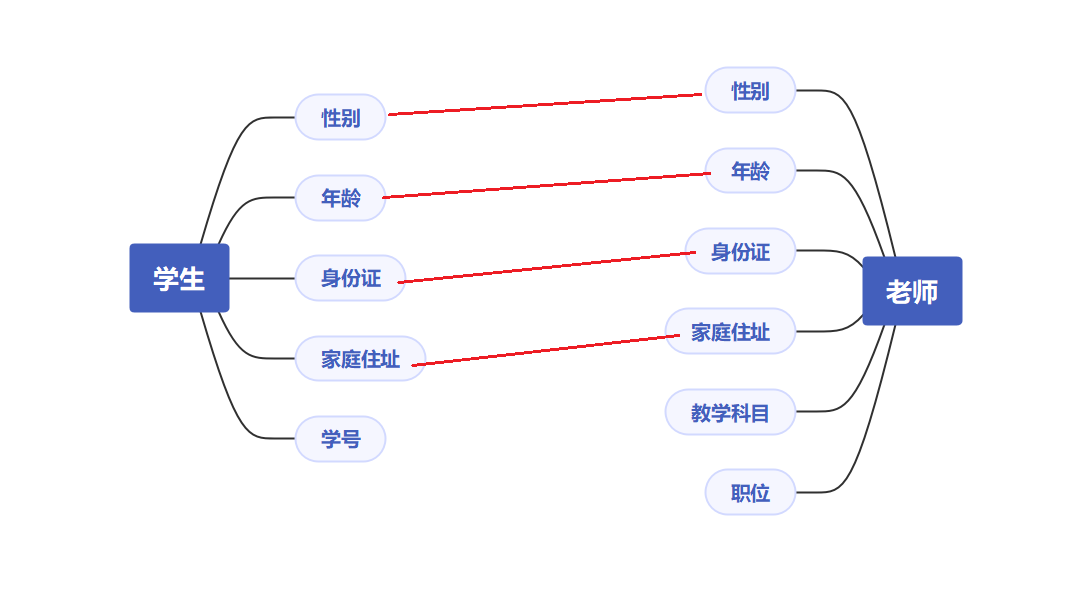``````//基类(父类)
class Person
{
public:
void print()
{
cout << _age << endl;
}
protected:
string _name; // 姓名
int _age; // 年龄
};
//派生类(子类) /继承方式 /(基类)父类
class Student : public Person
{
protected:
string _number;//学号
};
class Teacher : public Person
{
protected:
string _position;//职称
};

int main()
{
Student s;
s.print();
Teacher t;
t.print();
}
``````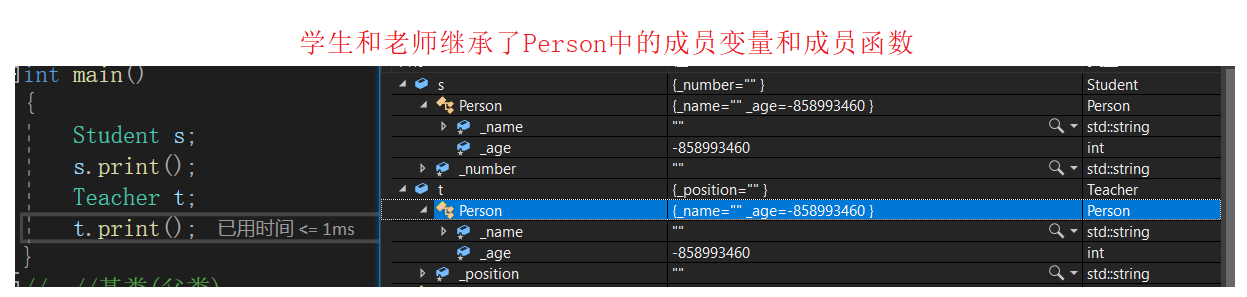这里Person称为父类，也称为基类，Student和Teacher称为子类，也称为派生类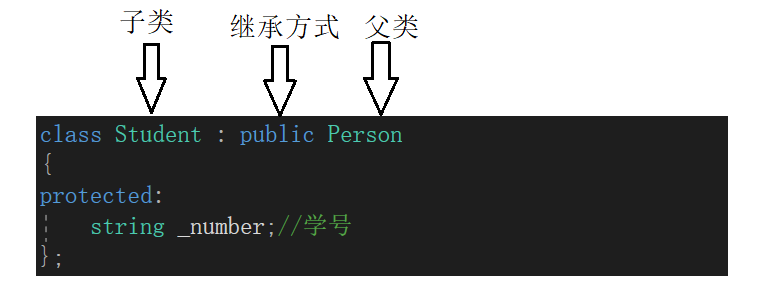# 🌌继承关系和访问限定符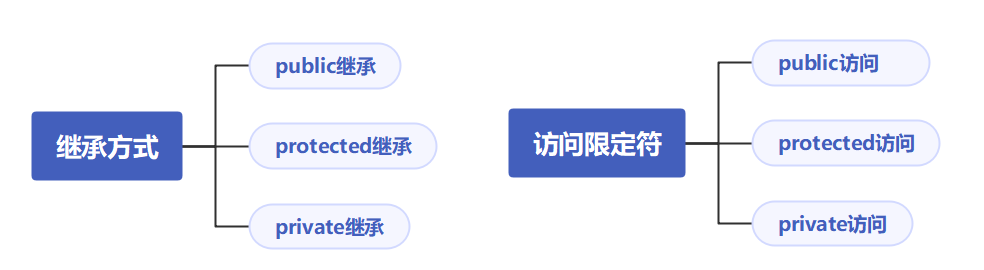• 基类的private成员在派生类中无论以什么方式继承都不可见，这里的不可见不是派生类没有继承，而是派生类对象不管是在类里面还是类外都无法访问
• 基类的其他成员在派生类的访问方式 == Min(成员在基类的访问限定符，继承方式)，权限：public > protected > private

• 关键字class默认的继承方式是private，关键字struct默认的继承方式是public，和访问限定符类似
• 实际中一般都用public继承，且访问限定符一般用public和protected，因为在没有继承时，访问限定符private和protected作用类似，但有继承后，如果基类是private成员，那不管以什么方式继承，在派生类中都不可见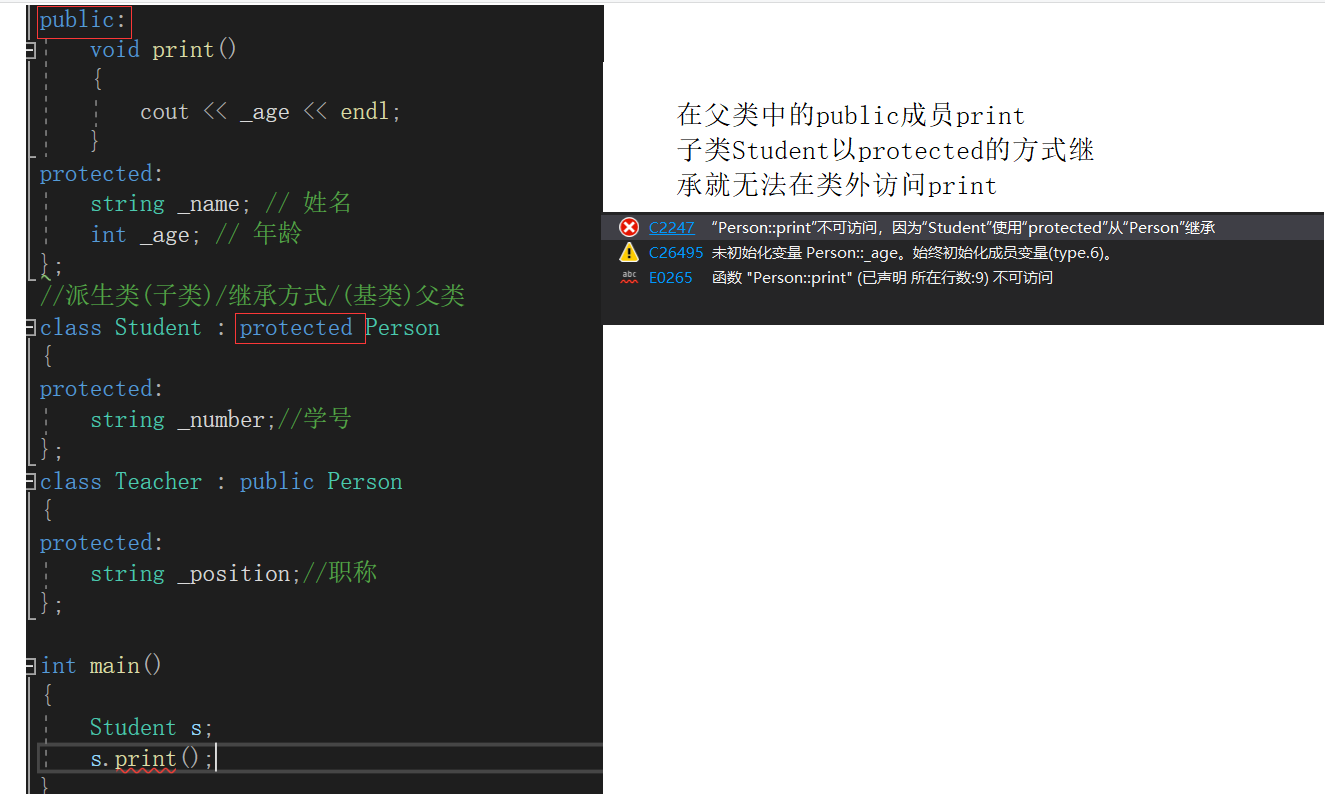# 🌌基类和派生类的赋值转换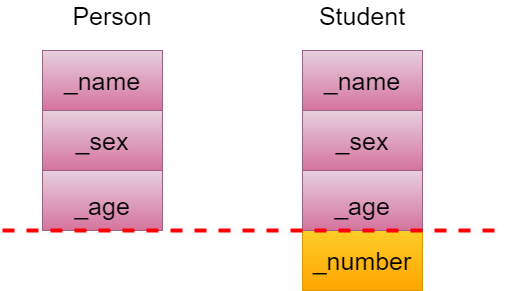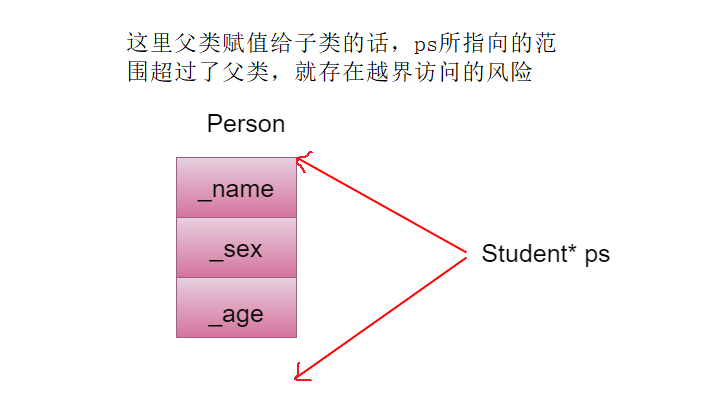``````class Person
{
protected:
string _name; // 姓名
string _sex;//性别
int _age; // 年龄
};
class Student : public Person
{
private:
string _number;//学号
};
int main()
{
Person p;
Student s;
//父类=子类，赋值兼容，叫切片，不存在类型转换，是天然的
p = s;
Person* ptr = &s;
Person& ref = s;

//子类=父类只能强转
Student* ps=(Student*)& p;
Student& st=(Student&)p;
return 0;
}
``````

# 🌌继承中的作用域

• 子类和父类都有同名的成员，子类将屏蔽对父类同名成员的直接访问（访问父类的隐藏成员时需要加访问限定符），叫做隐藏也叫重定义
• 如果是成员函数的隐藏，只需要函数名相同就构成隐藏，参数可相同也可不相同
``````class A
{
public:
void func()
{
cout << "func()" << endl;
}
};
class B : public A
{
public:
void func(int i)
{
A::func();
cout << "func(int i)->" << i << endl;
}
};
void Test1()
{
B b;
b.fun(10);
};
void Test2()
{
B b;
b.fun();
}
``````

``````b.A::fun();//指定作用域调父类的fun
``````

# 🌌派生类的默认成员函数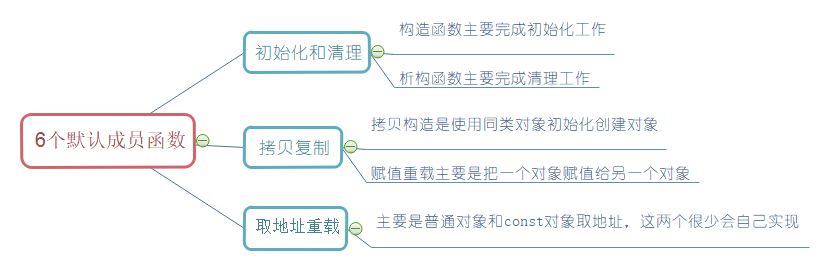1. 从父类继承下来的（调用父类的默认构造和析构）
2. 子类中自己的（对内置类型不处理，对自定义类型调用它的默认构造和析构）

1. 从父类继承下来的（调用父类的默认拷贝构造和operator=）
2. 自己的（对内置类型完成浅拷贝，对自定义类型调用它的默认拷贝构造和operator=）
 总结：从父类继承下来的调用父类的处理，自己的按照普通类处理
``````class Person
{
public:
Person(const char* name = "Bob")
: _name(name)
{
cout << "Person()" << endl;
}

Person(const Person& p)
: _name(p._name)
{
cout << "Person(const Person& p)" << endl;
}

Person& operator=(const Person& p)
{
cout << "Person operator=(const Person& p)" << endl;
if (this != &p)
_name = p._name;

return *this;
}
~Person()
{
cout << "~Person()" << endl;
}
protected:
string _name; // 姓名
};

class Student : public Person
{
public:
Student(const char* name = "peter", int num = 1)
:Person(name)//利用匿名对象调用父类的构造函数初始化
,_num(num)
{}
Student(const Student& s)
:Person(s)//调用父类的拷贝构造
,_num(s._num)
{
cout << "Student(const Student&)" << endl;
}
Student& operator=(const Student& s)
{
if (this != &s)
{
Person::operator=(s);//切片
_num = s._num;
}
return *this;
}
~Student()
{
cout << "~Student()" << endl;
}
protected:
int _num; //学号
};
int main()
{
Student s1;
Student s2(s1);
Student s3("Bob", 20);
s2 = s3;

return 0;
}
``````

• 由于调用构造和析构的时机与栈后进先出的原则类似，`先构造的后析构``后构造的先析构`，当我们创建子类对象时，父类先构造，之后才构造子类自己的，所以析构的时候父类需要后析构，如果父类析构的调用交给我们完成的话可能会影响调用顺序，所以父类的析构是交给编译器完成的，所以在子类的析构函数中不需要我们显示调用父类的析构函数。
• ps：就算要手动调用父类的析构函数，也需要加访问限定符指定作用域，因为`析构函数名\~Person()和~Student()会被统一处理成destructor()`，所以子类和父类的函数名相同就`构成隐藏`,所以需要指定作用域
``````~Student()
{
Person::~Person();
cout << "~Student()" << endl;
}
``````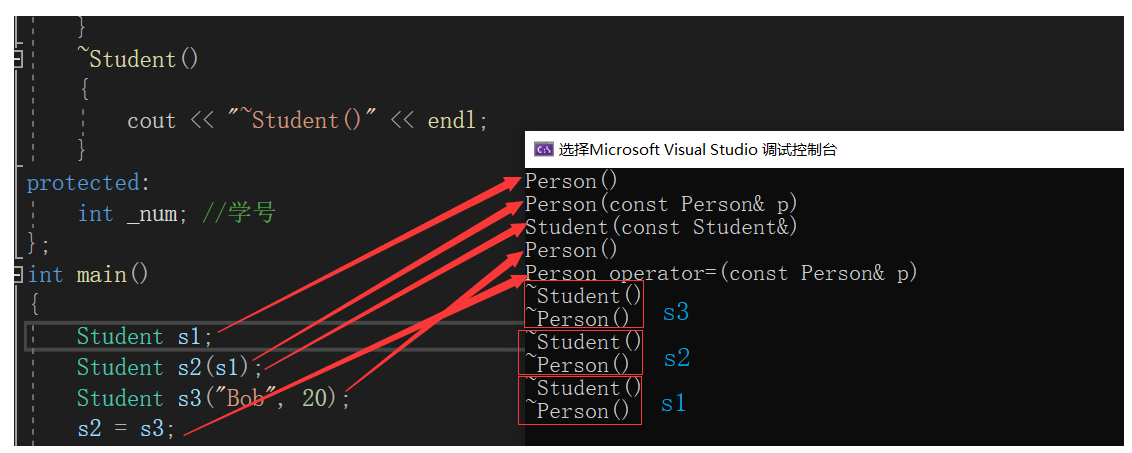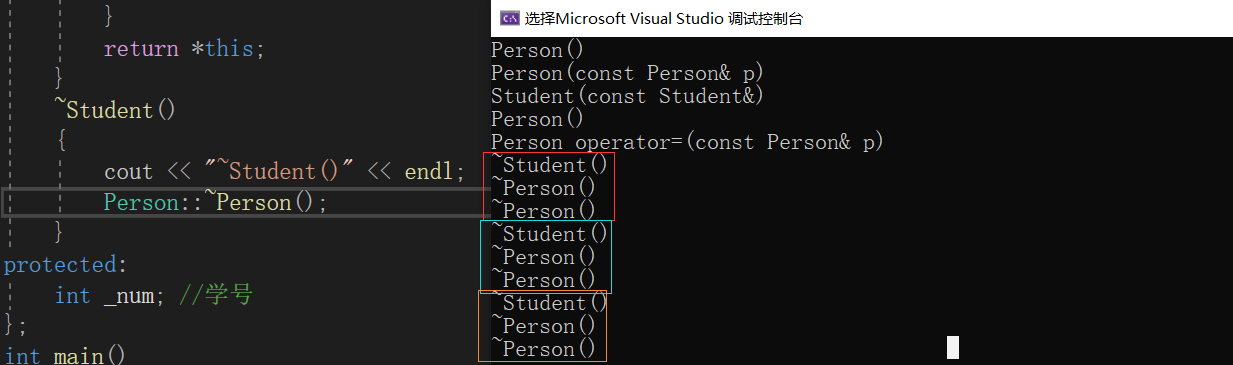# 🪐继承和友元&&继承和静态成员

Display虽然是Person的友元，虽然Student继承了Person，但Display不是Student的友元，所以这里是无法调用s中的_stuNum的

``````class Person
{
public:
friend void Display(const Person& p, const Student& s);
protected:
string _name; // 姓名
};
class Student : public Person
{
protected:
int _stuNum; // 学号
};
void Display(const Person& p, const Student& s)
{
cout << p._name << endl;
cout << s._stuNum << endl;
}
int main()
{
Person p;
Student s;
Display(p);
}

``````

# 🪐菱形继承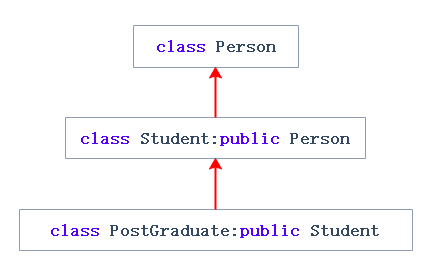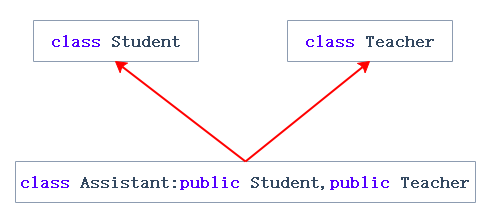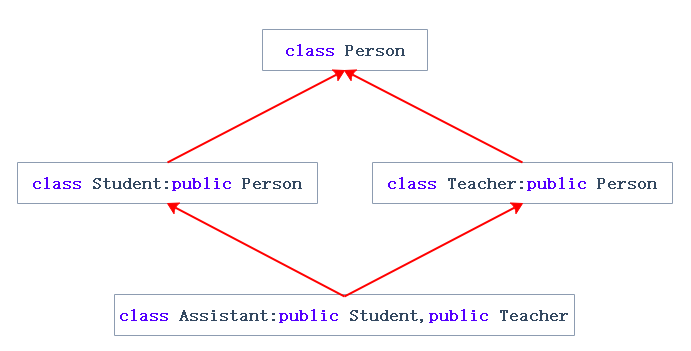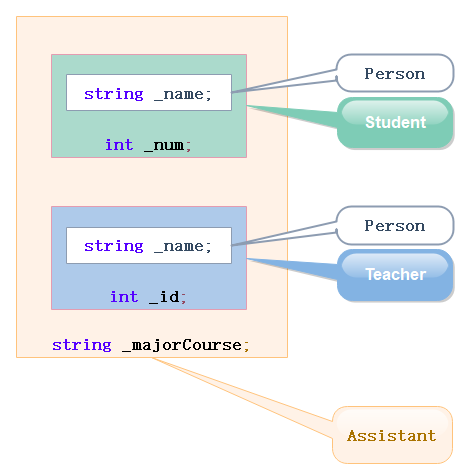``````class Person
{
public:
string _name; // 姓名
int _arr;
};

class Student : public Person
{
public:
int _num; //学号
};
class Teacher : public Person
{
public:
int _id; // 职工编号
};
class Assistant : public Student, public Teacher
{
public:
string _majorCourse; // 主修课程
};
``````

Assistant类有两份Person类即数据冗余，Assistant中就会有两个_name即二义性，这里指定_name后虽然可以消除二义性但是解决不了数据冗余问题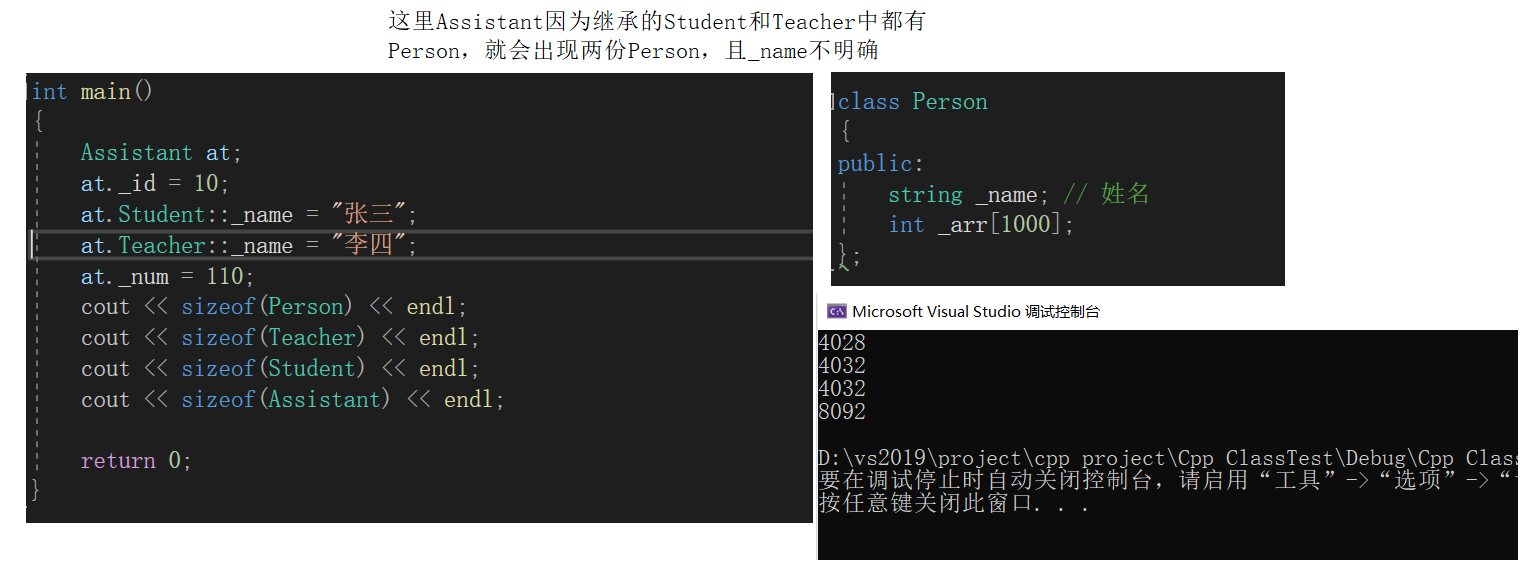# 🪐虚继承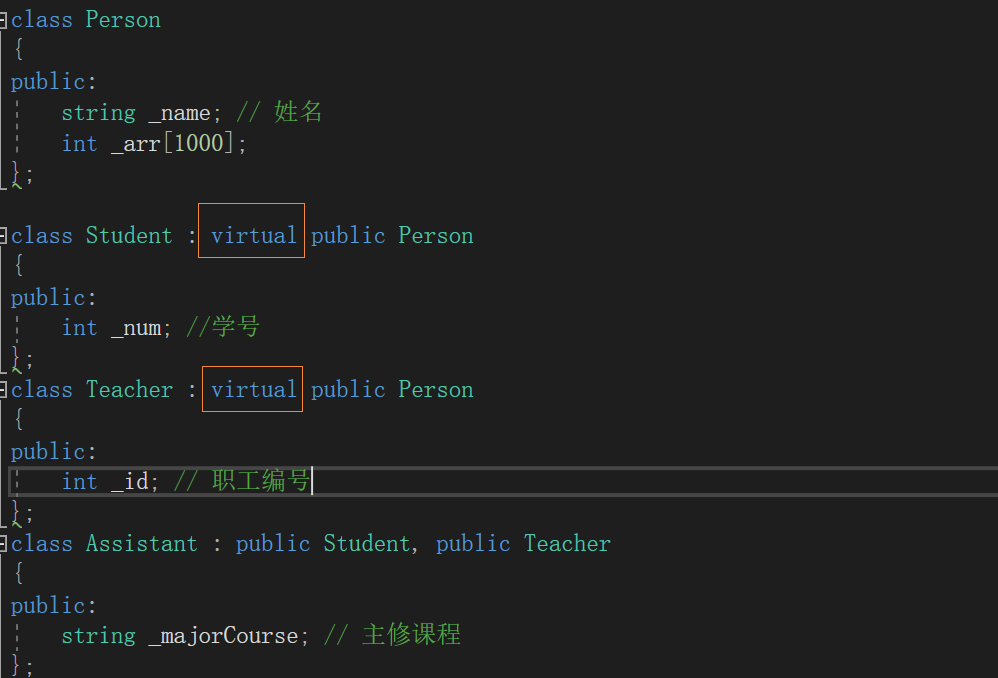ps：这也是菱形继承，是在B和D加virtual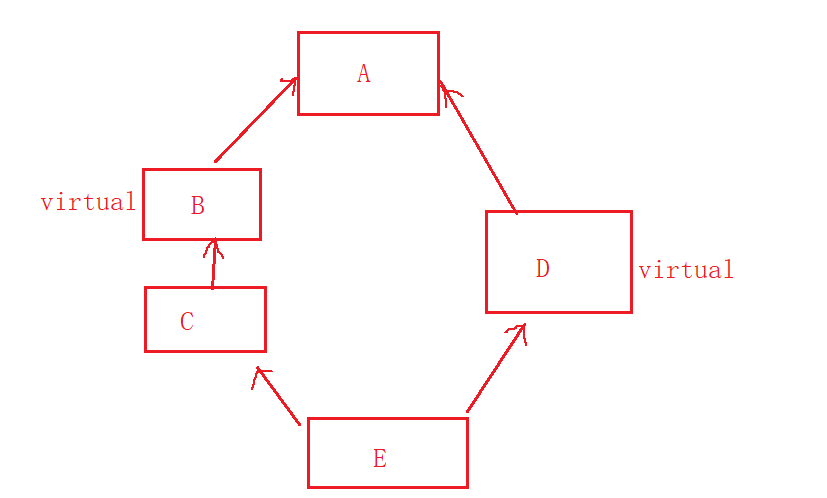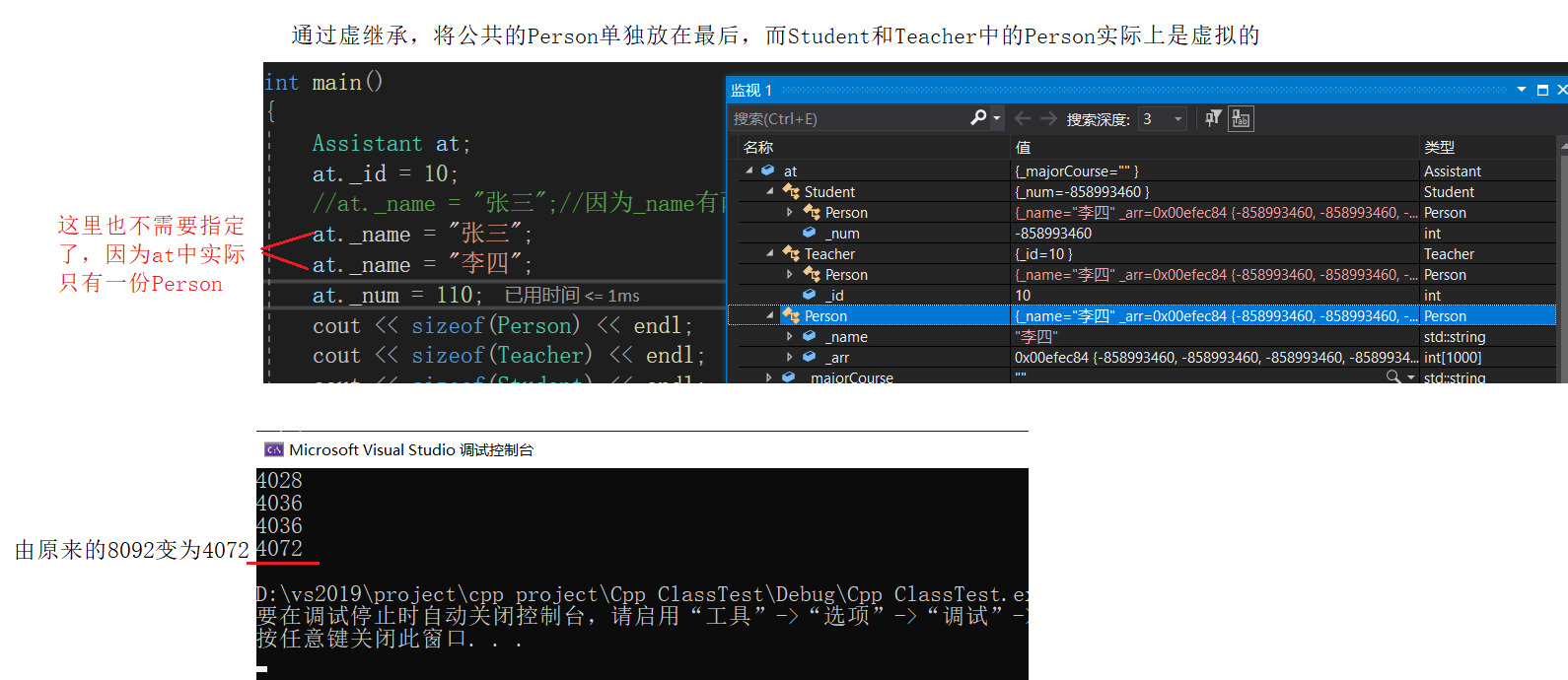## 🌏虚继承原理

``````class A
{
public:
int _a;
};
class B : public A
//class B : virtual public A
{
public:
int _b;
};
class C : public A
//class C : virtual public A
{
public:
int _c;
};
class D : public B, public C
{
public:
int _d;
};
int main()
{
D d;
d.B::_a = 1;
d.C::_a = 2;
d._b = 3;
d._c = 4;
d._d = 5;					//不加virtual时
cout << sizeof(A) << endl;	// _a
cout << sizeof(B) << endl;	//_a _b
cout << sizeof(C) << endl;	// _a _c
cout << sizeof(D) << endl;	//_a _a _b _c _d

return 0;
}
``````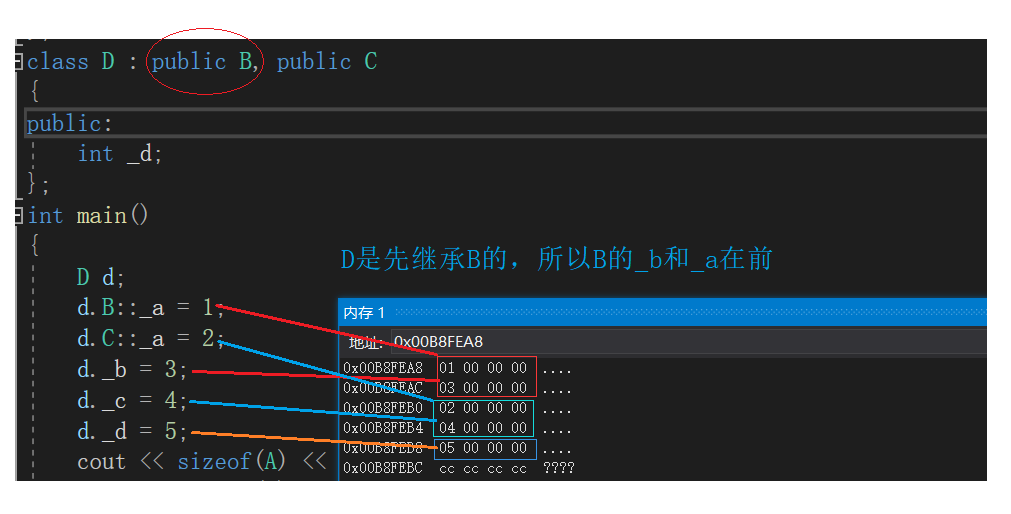采用虚继承后，d继承了B类中的_b和C类中的_c，然后将A类的_a(公共的)放在了最后。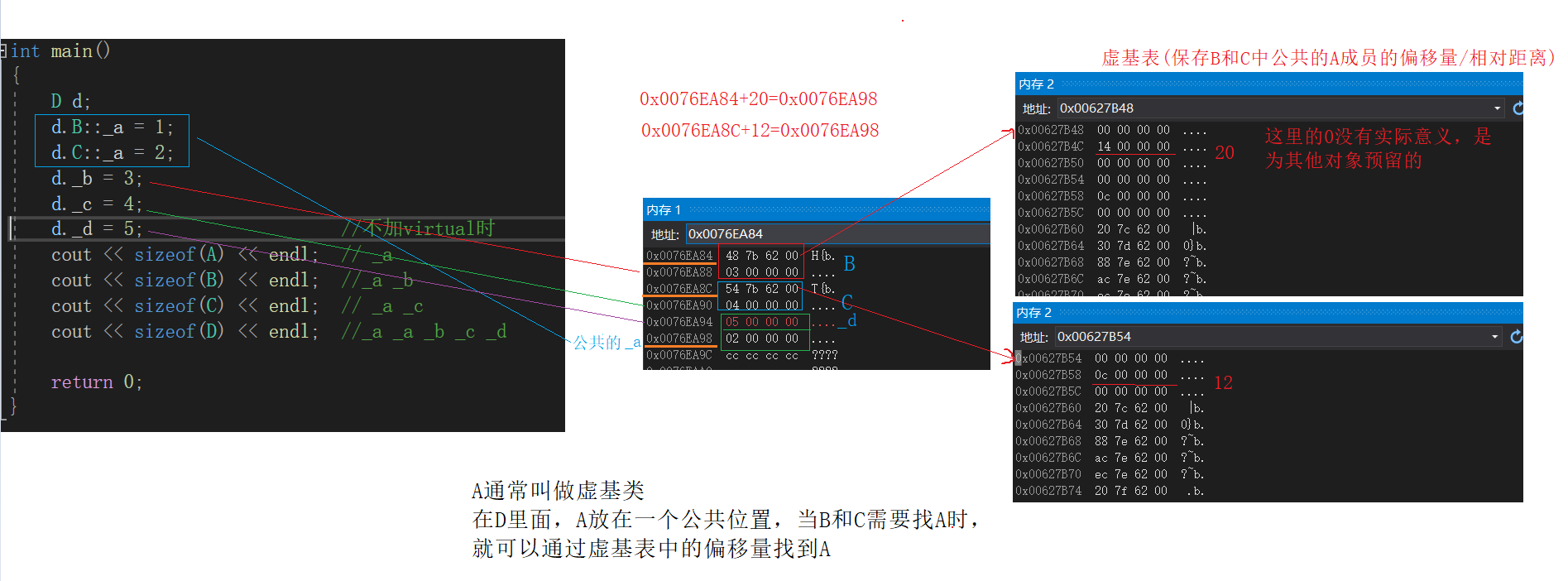# 🪐继承总结

• public继承是一种`is-a`的关系，也就是说每个派生类对象都是一个基类对象。比如Student继承Person，Student对象也是Person对象
• 组合是一种`has-a`的关系，B组合A，每个B对象都有一个A对象。比如汽车组合轮胎，汽车和轮胎就是has-a的关系
``````class A
{
private:
int _a;
};
//B和A是has-a关系
class B
{
private:
A _aa;
int _b;
};
``````

• 使用类继承，派生类和基类的依赖关系很强，耦合度高，改变基类就会影响派生类
• 而组合类之间没有很强的依赖关系，耦合度底。

# 🪐常见笔试面试题

1. 什么是菱形继承？菱形继承的问题是什么？
2. 什么是菱形虚拟继承？如何解决数据冗余和二义性的
3. 继承和组合的区别？什么时候用继承？什么时候用组合？

1️⃣菱形继承是特殊的多继承，当多继承中有公共的祖先时就是菱形继承。菱形继承的问题就是数据冗余和二义性

2️⃣菱形虚拟继承是解决菱形继承存在问题的一种继承方式，在直接继承公共祖先的两个类加上关键字virtual构成虚拟继承。正因为有公共祖先所以存在继承多份祖先类的问题，也就存在数据冗余和二义性，所以将祖先类放在一个公共位置，派生类存放当了对应的虚基表地址，虚基表中存放了当前位置相对祖先类的偏移量，根据偏移量就可以找到祖先类，从而解决了数据冗余和二义性的问题

3️⃣第三点在继承总结已经详细说明了